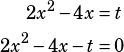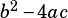Test 6 Section 3 #13

I’ll give you two ways to think about this one. Both begin with taking the given equation and putting it in standard form:The quick and dirty way to go from there (my preferred method because I’m all about graphs) is to recognize that this is a parabola that opens upwards (the first coefficient of positive 2 tells us that) and has a y-intercept of –t. Because a parabola has no real solutions when it doesn’t cross the x-axis, and because only one of the four choices can be correct, the highest y-intercept must be the answer. That will happen when –t is the greatest, which will happen when t is the least. When t = –3, then –t = –(–3) = 3. We’re not going to do better than that, so choice A is the answer.

The mathier way to go is to is to use the discriminant (AKA the part under the square root sign in the quadratic formula). When the discriminant is negative, there are no real solutions.

The discriminant is. In this case, that’s. Of the choices, which value of t makes the discriminant negative? Only choice A, –3, does.